# Digital Image Processing Image Restoration and Reconstruction Image

• Slides: 41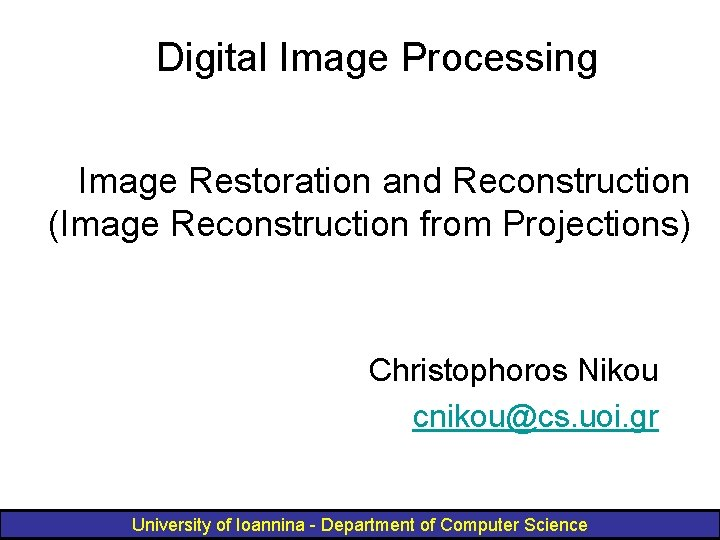Digital Image Processing Image Restoration and Reconstruction (Image Reconstruction from Projections) Christophoros Nikou [email protected] uoi. gr University of Ioannina - Department of Computer Science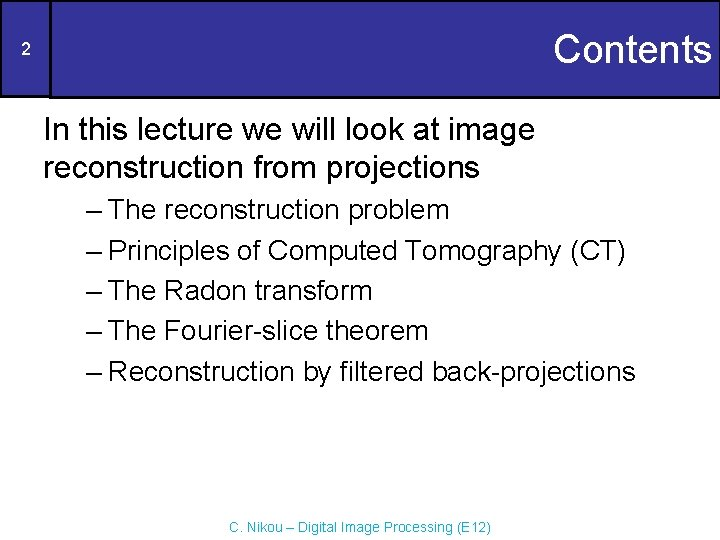Contents 2 In this lecture we will look at image reconstruction from projections – The reconstruction problem – Principles of Computed Tomography (CT) – The Radon transform – The Fourier-slice theorem – Reconstruction by filtered back-projections C. Nikou – Digital Image Processing (E 12)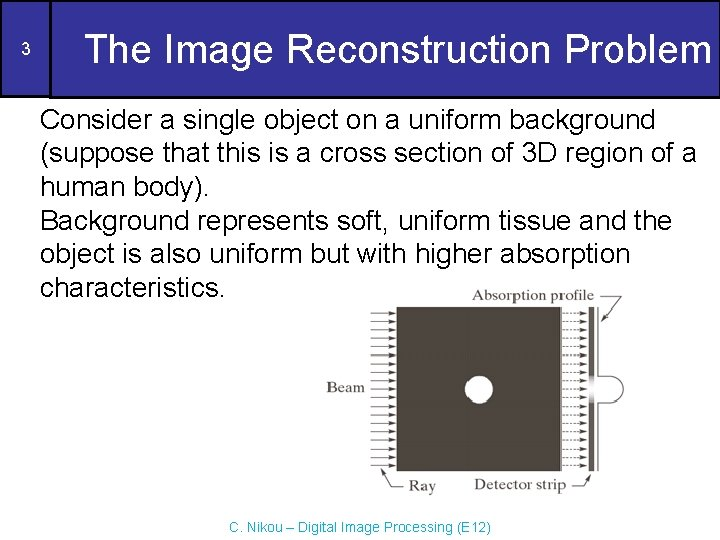3 The Image Reconstruction Problem Consider a single object on a uniform background (suppose that this is a cross section of 3 D region of a human body). Background represents soft, uniform tissue and the object is also uniform but with higher absorption characteristics. C. Nikou – Digital Image Processing (E 12)4 The Image Reconstruction Problem (cont. . . ) A beam of X-rays is emitted and part of it is absorbed by the object. The energy of absorption is detected by a set of detectors. The collected information is the absorption signal. C. Nikou – Digital Image Processing (E 12)5 The Image Reconstruction Problem (cont. . . ) A simple way to recover the object is to back-project the 1 D signal across the direction the beam came. This simply means to duplicate the signal across the 1 D beam. C. Nikou – Digital Image Processing (E 12)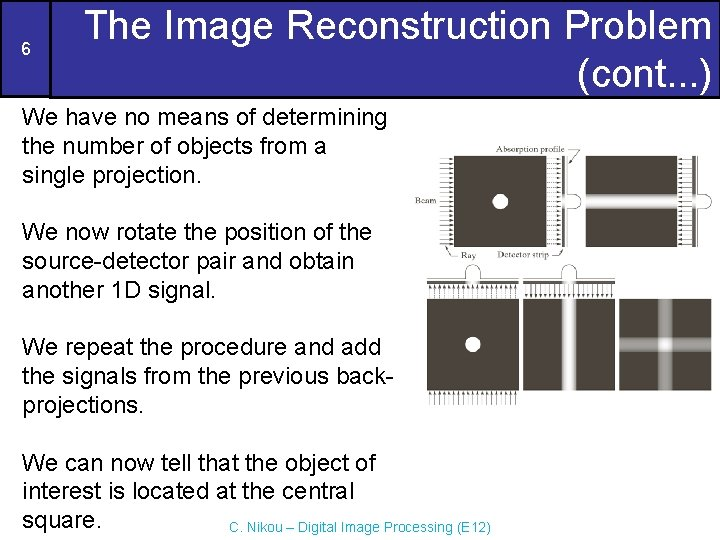6 The Image Reconstruction Problem (cont. . . ) We have no means of determining the number of objects from a single projection. We now rotate the position of the source-detector pair and obtain another 1 D signal. We repeat the procedure and add the signals from the previous backprojections. We can now tell that the object of interest is located at the central square. C. Nikou – Digital Image Processing (E 12)7 The Image Reconstruction Problem (cont. . . ) By taking more projections: • the form of the object becomes clearer as brighter regions will dominate the result • back-projections with few interactions with the object will fade into the background. C. Nikou – Digital Image Processing (E 12)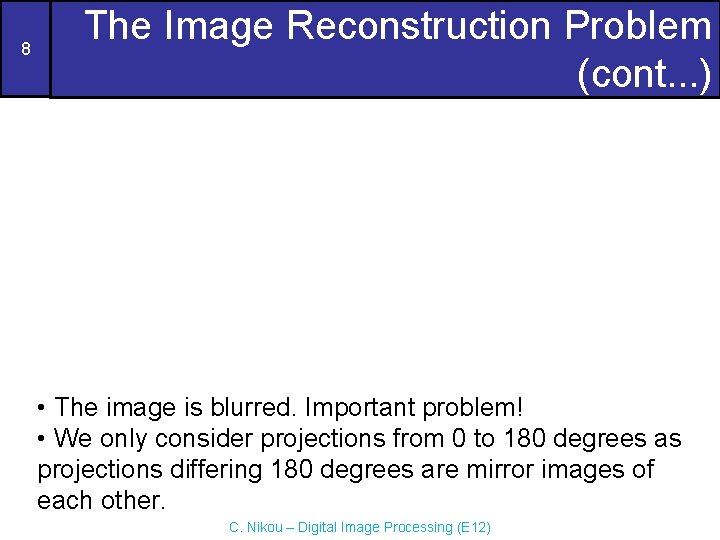8 The Image Reconstruction Problem (cont. . . ) • The image is blurred. Important problem! • We only consider projections from 0 to 180 degrees as projections differing 180 degrees are mirror images of each other. C. Nikou – Digital Image Processing (E 12)9 The Image Reconstruction Problem (cont. . . ) C. Nikou – Digital Image Processing (E 12)10 Principles of Computerized Tomography The goal of CT is to obtain a 3 D representation of the internal structure of an object by X-raying it from many different directions. Imagine the traditional chest X-ray obtained by different directions. The image is the 2 D equivalent of a line projections. Back-projecting the image would result in a 3 D volume of the chest cavity. C. Nikou – Digital Image Processing (E 12)11 Principles of Computerized Tomography (cont. . . ) CT gets the same information by generating slices through the body. A 3 D representation is then obtained by stacking the slices. More economical due to fewer detectors. Computational burden and dosage is reduced. Theory developed in 1917 by J. Radon. Application developed in 1964 by A. M. Cormack and G. N. Hounsfield independently. They shared the Nobel prize in Medicine in 1979. C. Nikou – Digital Image Processing (E 12)12 Principles of Computerized Tomography (cont. . . ) C. Nikou – Digital Image Processing (E 12)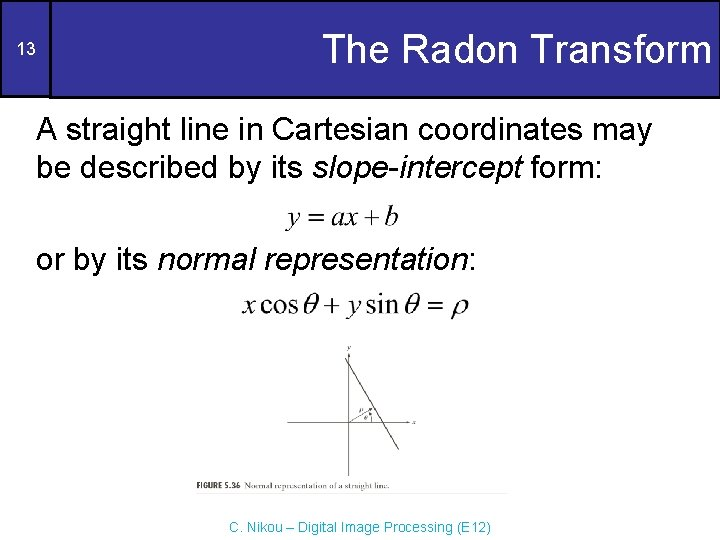13 The Radon Transform A straight line in Cartesian coordinates may be described by its slope-intercept form: or by its normal representation: C. Nikou – Digital Image Processing (E 12)14 The Radon Transform (cont. . . ) The projection of a parallel-ray beam may be modelled by a set of such lines. An arbitrary point (ρj, θk) in the projection signal is given by the ray-sum along the line xcosθk+ysinθk=ρj. C. Nikou – Digital Image Processing (E 12)15 The Radon Transform (cont. . . ) The ray-sum is a line integral: C. Nikou – Digital Image Processing (E 12)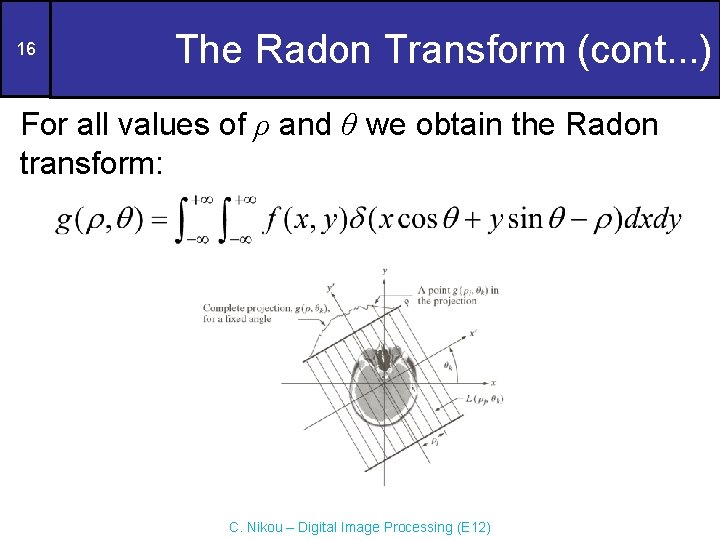16 The Radon Transform (cont. . . ) For all values of ρ and θ we obtain the Radon transform: C. Nikou – Digital Image Processing (E 12)17 The Radon Transform (cont. . . ) The representation of the Radon transform g(ρ, θ) as an image with ρ and θ as coordinates is called a sinogram. It is very difficult to interpret a sinogram. C. Nikou – Digital Image Processing (E 12)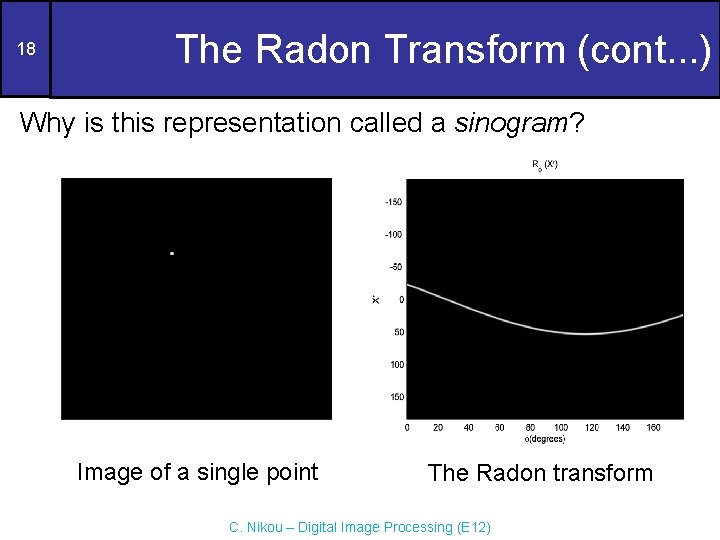18 The Radon Transform (cont. . . ) Why is this representation called a sinogram? Image of a single point The Radon transform C. Nikou – Digital Image Processing (E 12)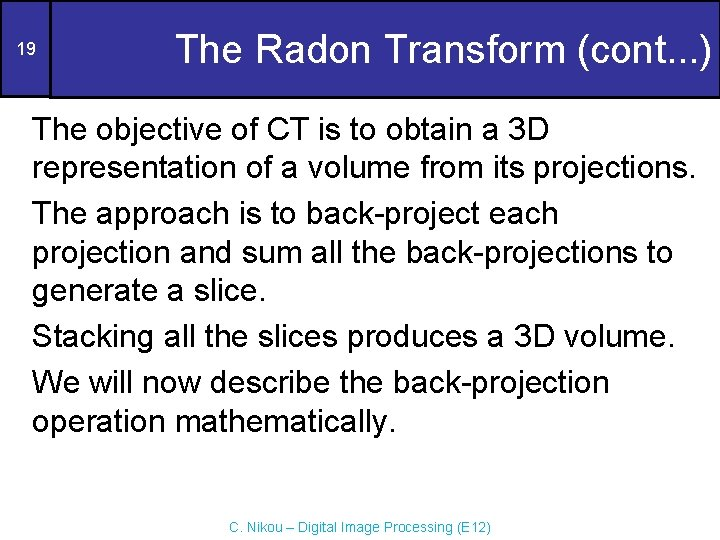19 The Radon Transform (cont. . . ) The objective of CT is to obtain a 3 D representation of a volume from its projections. The approach is to back-project each projection and sum all the back-projections to generate a slice. Stacking all the slices produces a 3 D volume. We will now describe the back-projection operation mathematically. C. Nikou – Digital Image Processing (E 12)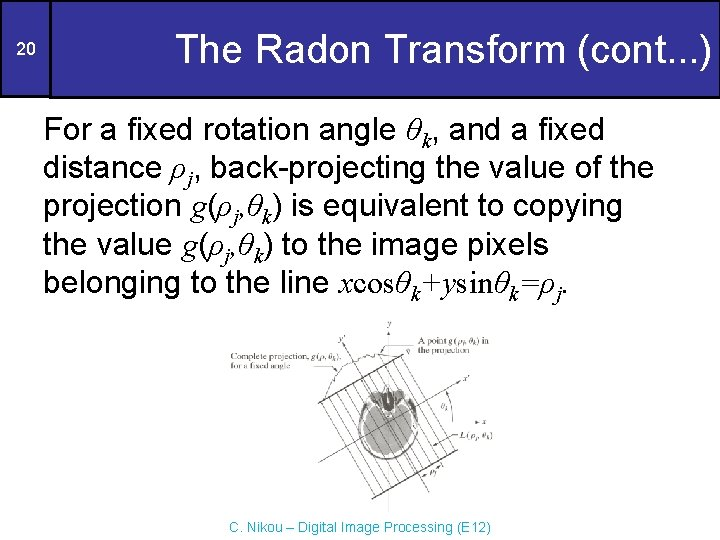20 The Radon Transform (cont. . . ) For a fixed rotation angle θk, and a fixed distance ρj, back-projecting the value of the projection g(ρj, θk) is equivalent to copying the value g(ρj, θk) to the image pixels belonging to the line xcosθk+ysinθk=ρj. C. Nikou – Digital Image Processing (E 12)21 The Radon Transform (cont. . . ) Repeating the process for all values of ρj, having a fixed angle θk results in the following expression for the image values: This equation holds for every angle θ: C. Nikou – Digital Image Processing (E 12)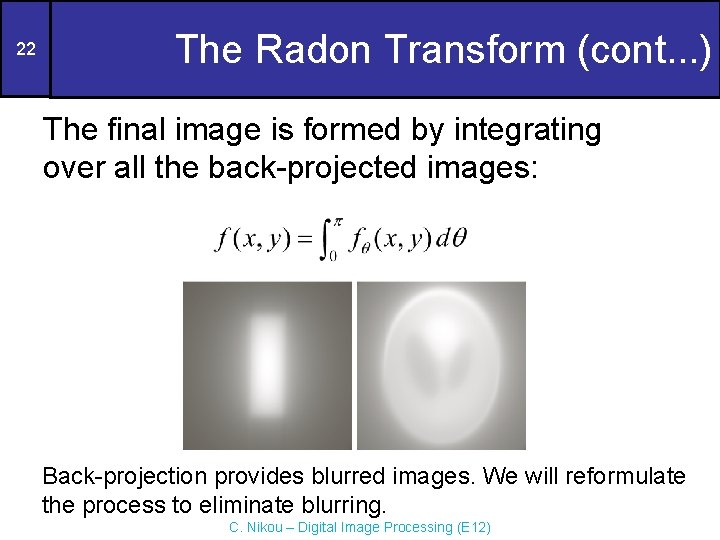22 The Radon Transform (cont. . . ) The final image is formed by integrating over all the back-projected images: Back-projection provides blurred images. We will reformulate the process to eliminate blurring. C. Nikou – Digital Image Processing (E 12)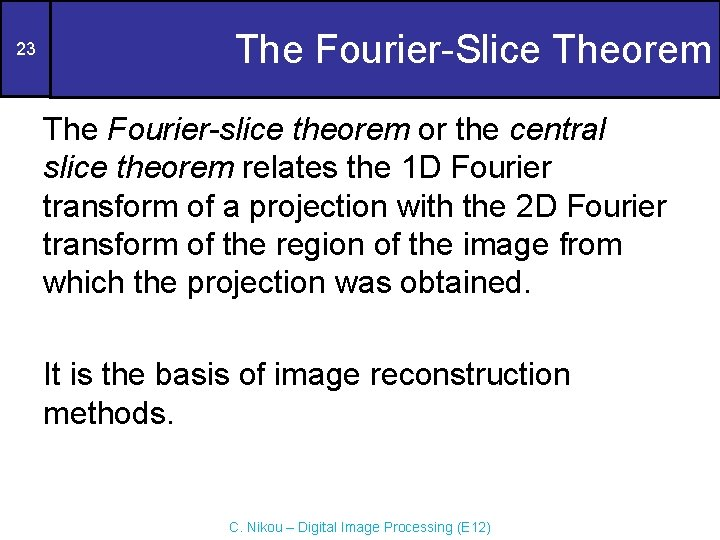23 The Fourier-Slice Theorem The Fourier-slice theorem or the central slice theorem relates the 1 D Fourier transform of a projection with the 2 D Fourier transform of the region of the image from which the projection was obtained. It is the basis of image reconstruction methods. C. Nikou – Digital Image Processing (E 12)24 The Fourier-Slice Theorem (cont. . . ) Let the 1 D F. T. of a projection with respect to ρ (at a given angle) be: Substituting the projection g(ρ, θ) by the ray-sum: C. Nikou – Digital Image Processing (E 12)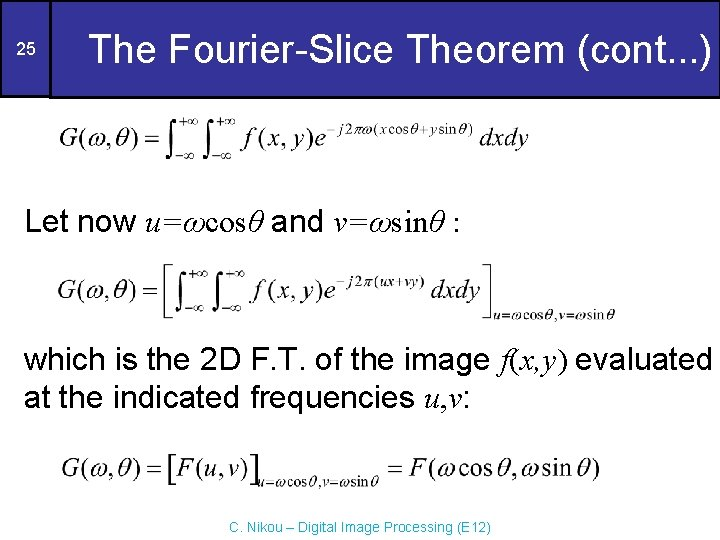25 The Fourier-Slice Theorem (cont. . . ) Let now u=ωcosθ and v=ωsinθ : which is the 2 D F. T. of the image f(x, y) evaluated at the indicated frequencies u, v: C. Nikou – Digital Image Processing (E 12)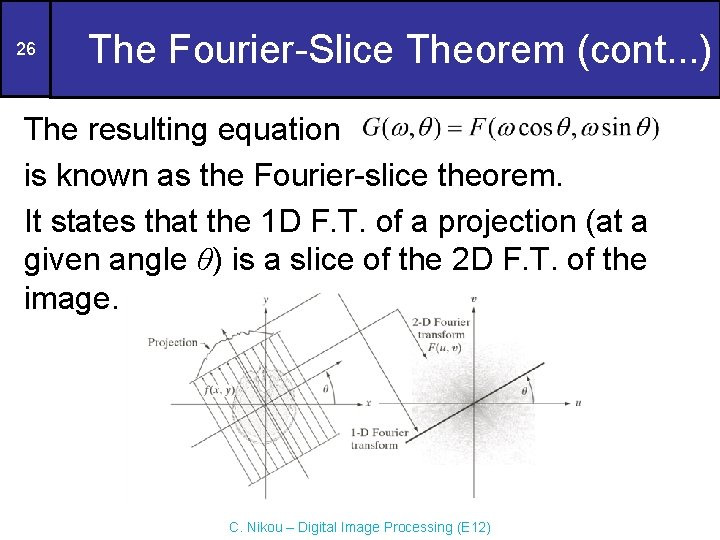26 The Fourier-Slice Theorem (cont. . . ) The resulting equation is known as the Fourier-slice theorem. It states that the 1 D F. T. of a projection (at a given angle θ) is a slice of the 2 D F. T. of the image. C. Nikou – Digital Image Processing (E 12)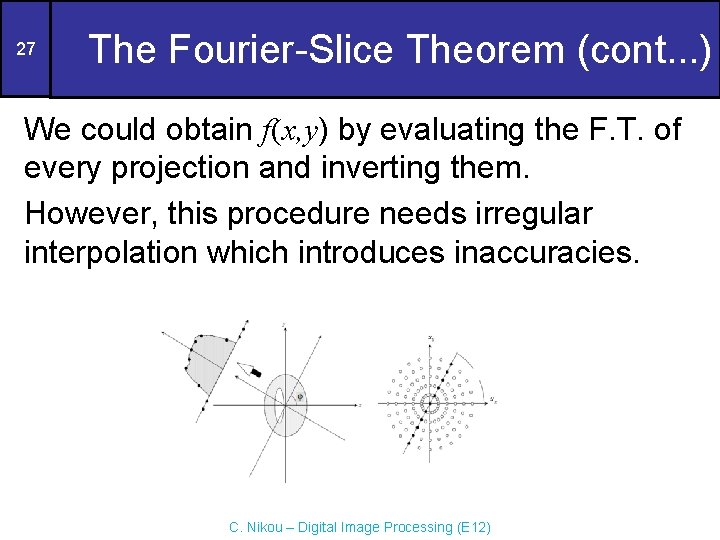27 The Fourier-Slice Theorem (cont. . . ) We could obtain f(x, y) by evaluating the F. T. of every projection and inverting them. However, this procedure needs irregular interpolation which introduces inaccuracies. C. Nikou – Digital Image Processing (E 12)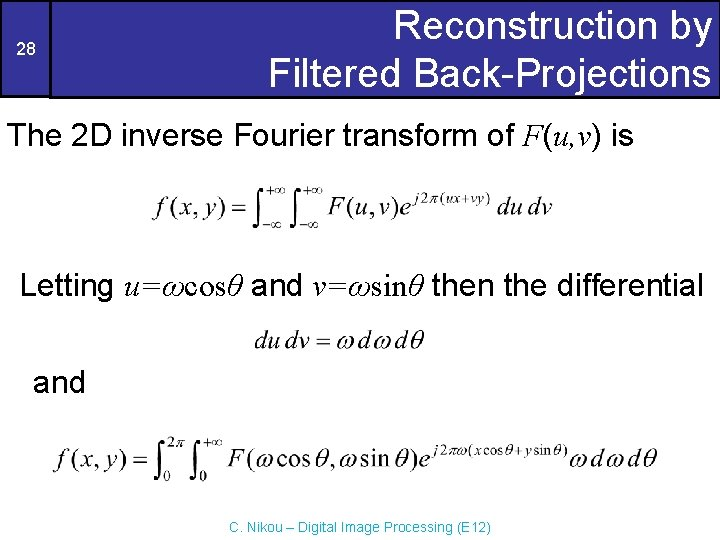28 Reconstruction by Filtered Back-Projections The 2 D inverse Fourier transform of F(u, v) is Letting u=ωcosθ and v=ωsinθ then the differential and C. Nikou – Digital Image Processing (E 12)29 Reconstruction by Filtered Back-Projections (cont. . . ) Using the Fourier-slice theorem: With some manipulation (left as an exercise, see the textbook): The term xcosθ+ysinθ=ρ and is independent of ω: C. Nikou – Digital Image Processing (E 12)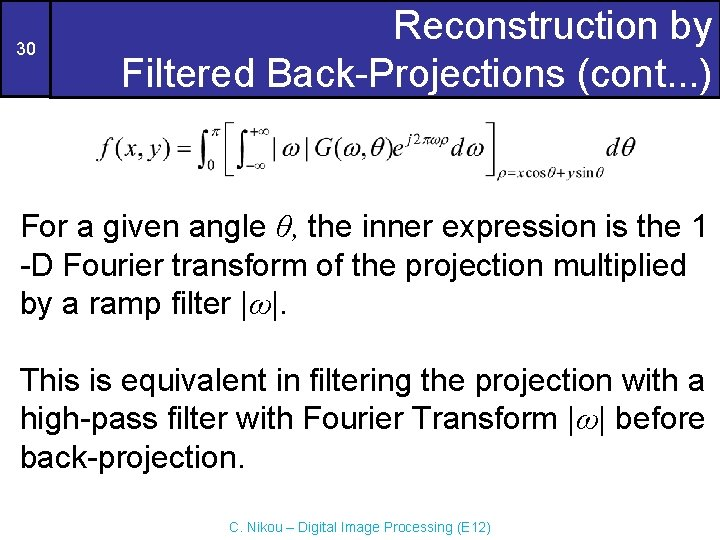30 Reconstruction by Filtered Back-Projections (cont. . . ) For a given angle θ, the inner expression is the 1 -D Fourier transform of the projection multiplied by a ramp filter |ω|. This is equivalent in filtering the projection with a high-pass filter with Fourier Transform |ω| before back-projection. C. Nikou – Digital Image Processing (E 12)31 Reconstruction by Filtered Back-Projections (cont. . . ) Problem: the filter H(ω)=|ω| is not integrable in the inverse Fourier transform as it extends to infinity in both directions. It should be truncated in the frequency domain. The simplest approach is to multiply it by a box filter in the frequency domain. Ringing will be noticeable. Windows with smoother transitions are used. C. Nikou – Digital Image Processing (E 12)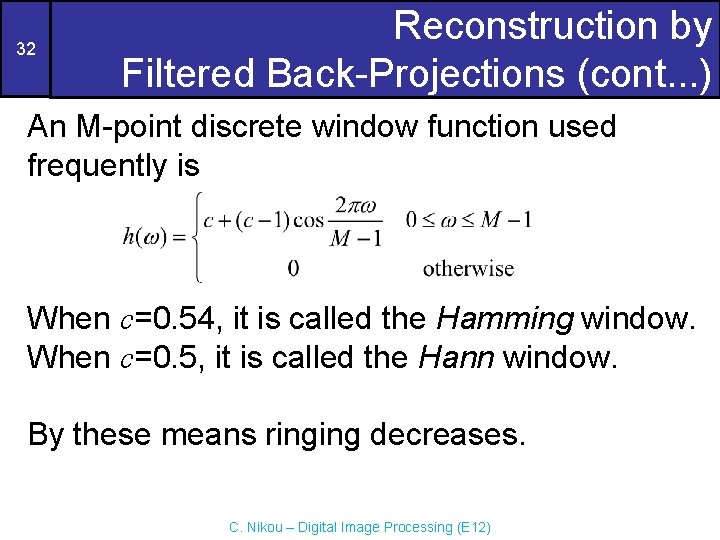32 Reconstruction by Filtered Back-Projections (cont. . . ) An M-point discrete window function used frequently is When c=0. 54, it is called the Hamming window. When c=0. 5, it is called the Hann window. By these means ringing decreases. C. Nikou – Digital Image Processing (E 12)33 Reconstruction by Filtered Back-Projections (cont. . . ) Ramp filter multiplied by a box window Hamming window Ramp filter multiplied by a Hamming window C. Nikou – Digital Image Processing (E 12)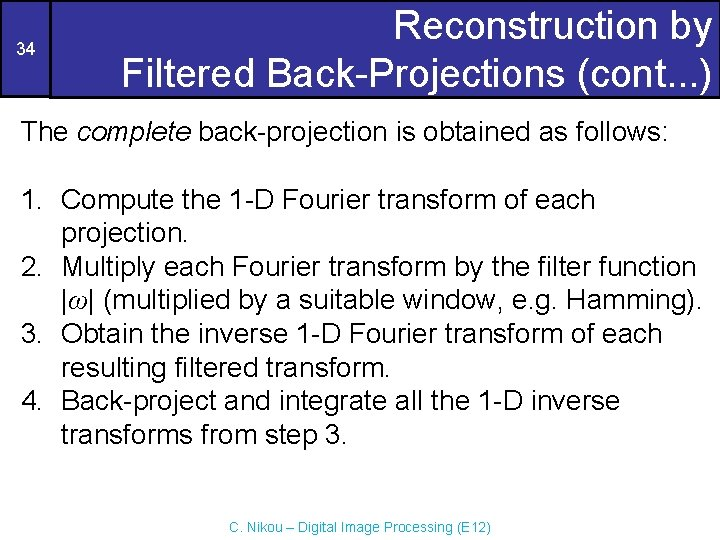34 Reconstruction by Filtered Back-Projections (cont. . . ) The complete back-projection is obtained as follows: 1. Compute the 1 -D Fourier transform of each projection. 2. Multiply each Fourier transform by the filter function |ω| (multiplied by a suitable window, e. g. Hamming). 3. Obtain the inverse 1 -D Fourier transform of each resulting filtered transform. 4. Back-project and integrate all the 1 -D inverse transforms from step 3. C. Nikou – Digital Image Processing (E 12)35 Reconstruction by Filtered Back-Projections (cont. . . ) Because of the filter function the reconstruction approach is called filtered back-projection (FBP). • Sampling issues must be taken into account to prevent aliasing. • The number of rays per angle which determines the number of samples for each projection. • The number of rotation angles which determines the number of reconstructed images. C. Nikou – Digital Image Processing (E 12)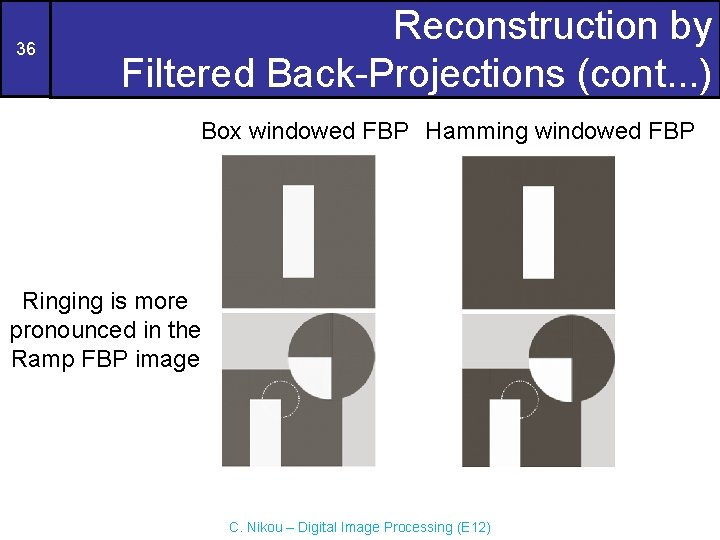36 Reconstruction by Filtered Back-Projections (cont. . . ) Box windowed FBP Hamming windowed FBP Ringing is more pronounced in the Ramp FBP image C. Nikou – Digital Image Processing (E 12)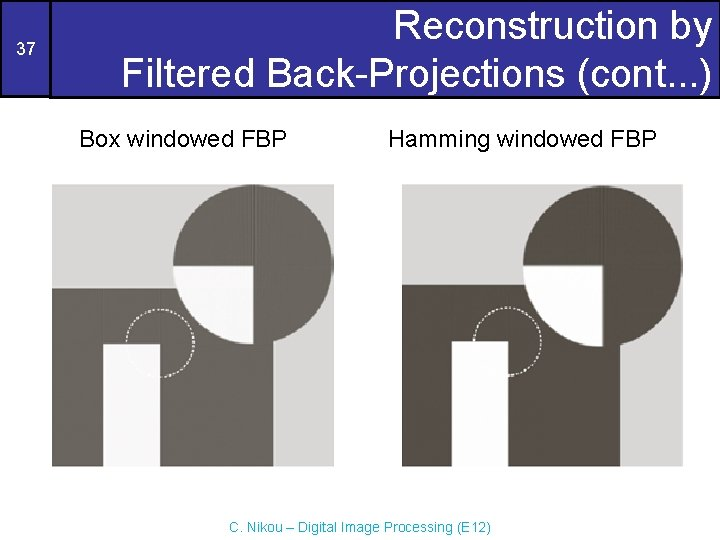37 Reconstruction by Filtered Back-Projections (cont. . . ) Box windowed FBP Hamming windowed FBP C. Nikou – Digital Image Processing (E 12)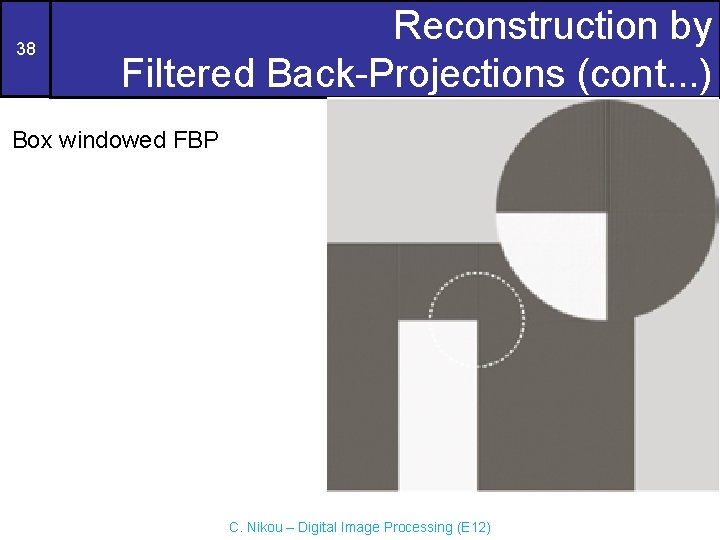38 Reconstruction by Filtered Back-Projections (cont. . . ) Box windowed FBP C. Nikou – Digital Image Processing (E 12)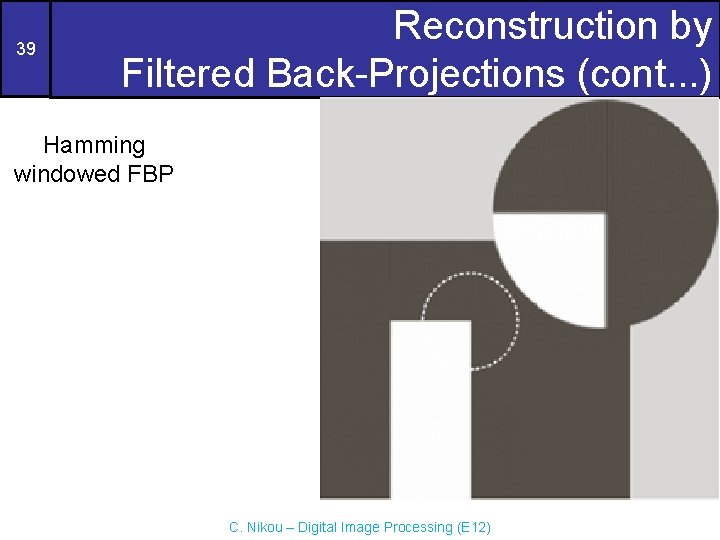39 Reconstruction by Filtered Back-Projections (cont. . . ) Hamming windowed FBP C. Nikou – Digital Image Processing (E 12)40 Reconstruction by Filtered Back-Projections (cont. . . ) Box windowed FBP Hamming windowed FBP There are no sharp transitions in the Shepp-Logan phantom and the two filters provide similar results. C. Nikou – Digital Image Processing (E 12)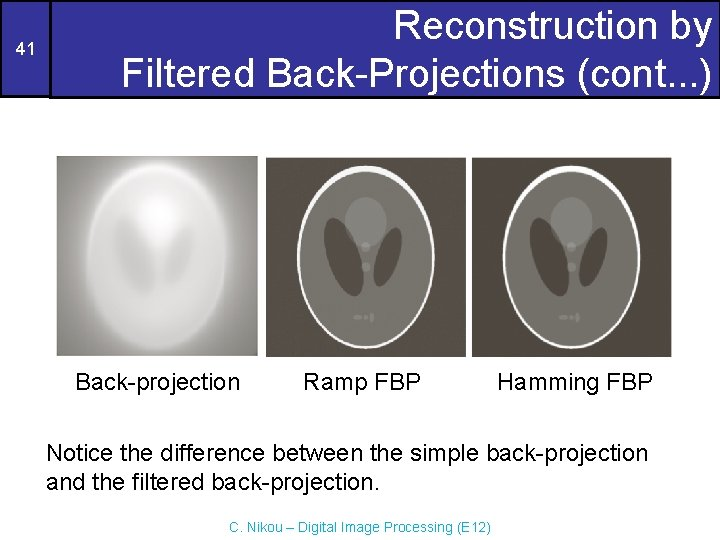41 Reconstruction by Filtered Back-Projections (cont. . . ) Back-projection Ramp FBP Hamming FBP Notice the difference between the simple back-projection and the filtered back-projection. C. Nikou – Digital Image Processing (E 12)